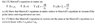# E&M Question -- Maxwell's Equation in matter reduce to Maxwell's equation in a vacuum....

ParticleGinger6
New user has been reminded to show their work on schoolwork questions
Homework Statement:
Maxwell's Equation in matter reduces to Maxwell's equation in vacuum if polarization and magnetization are zero?
Relevant Equations:
They can be found in the attached photo
I do not know where to start.

#### Attachments

•Chegg 5.6.20.JPG
33.5 KB · Views: 117

Homework Helper
Gold Member
How are polarization (i.e. the Polarization Vector Field) and magnetization (i.e. Magnetization Vector Field)\\ related to the vector fields $\vec D$ , $\vec E$, $\vec B$, and $\vec H$?

ParticleGinger6
@robphy so i found the equations D = epsilon*E and H = (1/mu)*H where epsilon = epsilon(not)*(1+Xe) and mu = mu(not)*(1+Xe). I think if I use that convert D into terms of P which would look like P = D - epsilon(not)*E and H = B/(mu(not)*(1+Xe)). From there you can get Magnetization from M = Xm*H

Am I on the right track

Yes... but use $\epsilon_0$ and $\mu_0$ instead of the $\chi$s.